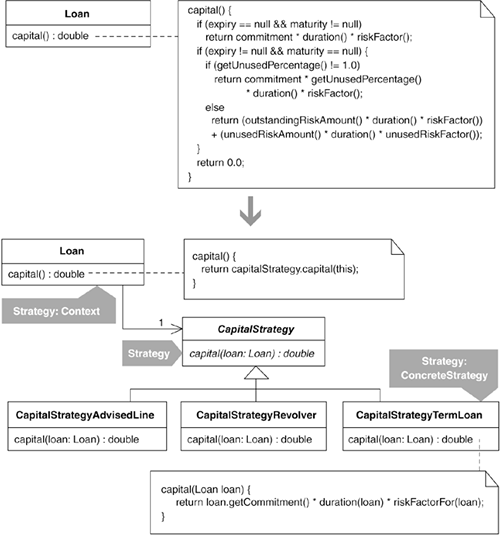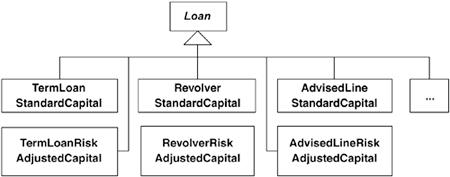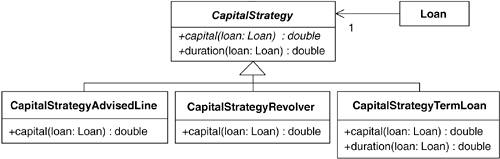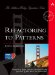# Replace Conditional Logic with Strategy

 Prev don't be afraid of buying books Next

### Replace Conditional Logic with Strategy

Conditional logic in a method controls which of several variants of a calculation are executed.

Create a Strategy for each variant and make the method delegate the calculation to a Strategy instance.#### Motivation

"Simplifying Conditional Expressions" is a chapter in Refactoring [F] that contains over a half-dozen highly useful refactorings devoted to cleaning up conditional complexity. About Decompose Conditional [F], Martin Fowler writes, "One of the most common areas of complexity in a program lies in complex conditional logic" [F, 238]. We often find such logic in algorithms because they tend to grow, becoming more sophisticated over time. The Strategy pattern [DP] helps manage the complexity that results from having numerous variations of an algorithm.

Conditional logic is often used to decide which variation of an algorithm to use. Refactorings like Decompose Conditional [F] or Compose Method (123) can simplify such code. On the other hand, they can also overrun your host class with small methods that apply only to specific variations of the algorithm, thereby complicating the host class. In such a situation, it may be better to move each algorithm variation to new classes or to subclasses of the host class. This essentially involves choosing between object composition and inheritance.

Replace Conditional Logic with Strategy (129) involves object composition: you produce a family of classes for each variation of the algorithm and outfit the host class with one Strategy instance to which the host delegates at runtime. An inheritance-based solution can be achieved by applying Replace Conditional with Polymorphism [F]. The prerequisite for that refactoring is an inheritance structure (i.e., the algorithm's host class must have subclasses). If subclasses exist and each variation of the algorithm maps easily to specific subclasses, this is probably your preferred refactoring. If you must first create the subclasses, you'll have to decide whether it would be easier to use an object-compositional approach, like refactoring to Strategy. If the conditionals in the algorithm are controlled by one type code, it may be easy to create subclasses of the algorithm's host class, one for each type code (see Replace Type Code with Subclasses [F]). If there is no such type code, you'll likely be better off refactoring to Strategy. Finally, if clients need to swap one calculation type for another at runtime, you'd better avoid an inheritance-based approach because this means changing the type of object a client is working with rather than simply substituting one Strategy instance for another.

When deciding whether to refactor to or towards a Strategy, you have to consider how the algorithm embedded in each Strategy will access the data it needs to do its job. As the Mechanics section points out, there are two ways to do this: pass the host class (called the context) to the Strategy so it can call back on methods to get its data, or just pass the data directly to the Strategy via parameters. Both of these approaches have upsides and downsides, which are discussed in the Mechanics section.

The Strategy and Decorator patterns offer alternative ways to eliminate conditional logic associated with special-case or alternative behavior. The sidebar Decorator vs. Strategy in the Motivation section of Move Embellishment to Decorator (144) offers a look at how these two patterns differ.

When implementing a Strategy-based design, you'll want to consider how your context class will obtain its Strategy. If you don't have many combinations of Strategies and context classes, it's good practice to shield client code from having to worry about both instantiating a Strategy instance and outfitting a context with a Strategy instance. Encapsulate Classes with Factory (80) can help with this: just define one or more methods that return a context instance, properly outfitted with the appropriate Strategy instance.

## Benefits and Liabilities

 + Clarifies algorithms by decreasing or removing conditional logic. + Simplifies a class by moving variations on an algorithm to a hierarchy. + Enables one algorithm to be swapped for another at runtime. – Complicates a design when an inheritance-based solution or a refactoring from "Simplifying Conditional Expressions" [F] is simpler. – Complicates how algorithms obtain or receive data from their context class.

#### Mechanics

Identify the context, a class with a calculation method that contains a lot of conditional logic.

1. Create a strategy, a concrete class (known as Strategy:ConcreteStrategy in Design Patterns [DP]) that will become a genuine ConcreteStrategy by the end of this refactoring. Name your strategy after the behavior performed by the calculation method. You can append the word "Strategy" to the class name if you find it helps communicate the purpose of this new type.

2. Apply Move Method [F] to move the calculation method to the strategy. When you perform this step, retain a version of the calculation method on the context that delegates to the strategy's calculation method. Implementing this delegation will involve defining and instantiating a delegate, a field in context that holds a reference to a strategy.

Since most strategies require data in order to do their calculations, you'll need to determine how to make that data available to the strategy. Here are two common approaches.

1. Pass the context as a parameter to the strategy's constructor or calculation method. This may involve making context methods public so the strategy can obtain the information it needs. A downside of this approach is that it often breaks "information hiding" (i.e., data that was visible only to the context now must be made visible to other classes, such as the strategy). An upside to this approach is that when you add new public methods to your context, they will be immediately available to all concrete strategies without a lot of code changes. If you take this approach, consider giving the least public access to context data—for example, in Java, consider giving your strategies package projection access to context data.

2. Pass the necessary data from the context to the strategy via calculation method parameters. A downside of this approach is that data will be passed to every concrete strategy regardless of whether particular strategies need that data. An upside to this approach is that it involves the least coupling between the context and the strategy.

A challenge of following this approach relates to the amount of data you'll need to pass to the strategy. If you have to pass ten parameters to your strategy, it may be better to pass the entire context as a reference to the strategy. On the other hand, you may be able to apply Introduce Parameter Object [F] to cut down the number of parameters you must pass to your strategy, thereby making data-passing an acceptable approach. Furthermore, if some parameters are passed to the strategy solely to handle the needs of a specific concrete strategy, you can remove that data from the parameter list and pass it to the concrete strategy via a constructor or initialization method.

There may be helper methods in the context that really belong in the strategy because they are referenced only by the calculation method that's now in the strategy. Move such helper methods from the context to the strategy by implementing whatever accessors are necessary.

• Compile and test.

3. Let clients outfit a context with an instance of the strategy by applying Extract Parameter (346) on the context code that instantiates a concrete strategy and assigns it to the delegate.

• Compile and test.

4. Apply Replace Conditional with Polymorphism [F] on the strategy's calculation method. To apply that refactoring, you'll first be given a choice to use Replace Type Code with Subclasses [F] or Replace Type Code with State/Strategy [F]. Choose the former. You'll need to implement Replace Type Code with Subclasses [F] whether you have an explicit type code or not. If conditional logic in the calculation method identifies particular types of the calculation, use that conditional logic in place of explicit types as you work through the mechanics for Replace Type Code with Subclasses [F].

Focus on producing one subclass at a time. Upon completion of this step, you'll have substantially reduced the conditional logic in the strategy and you'll have concrete strategies for each variety of the original calculation method. If possible, make the strategy an abstract class.

• Compile and test with combinations of context instances and concrete strategy instances.

#### Example

The example in the code sketch presented in the introduction to this refactoring deals with calculating capital for three different kinds of bank loans: a term loan, a revolver, and an advised line. It contains a fair amount of conditional logic used in performing the capital calculation, though it's less complicated and contains less conditional logic than was present in the original code, which handled capital calculations for seven distinct loan types.

In this example, we'll see how Loan's method for calculating capital can be strategized (i.e., delegated to a Strategy object). As you study the example, you may wonder why Loan wasn't simply subclassed to support the different styles of capital calculations. That would not have been a good design choice because the application that used Loan needed to accomplish the following.

• Calculate capital for loans in a variety of ways. Had there been one Loan subclass for each type of capital calculation, the Loan hierarchy would have been overburdened with subclasses, as shown in the diagram on the following page.• Change a loan's capital calculation at runtime, without changing the class type of the Loan instance. This is easier to do when it involves exchanging a Loan object's Strategy instance for another Strategy instance, rather than changing the whole Loan object from one subclass of Loan into another.

Now let's look at some code. The Loan class, which plays the role of the context (as defined in the Mechanics section), contains a calculation method called capital():

` public class Loan...    public double capital() {       if (expiry == null && maturity != null)          return commitment * duration() * riskFactor();       if (expiry != null && maturity == null) {          if (getUnusedPercentage() != 1.0)             return commitment * getUnusedPercentage() * duration() * riskFactor();          else             return (outstandingRiskAmount() * duration() * riskFactor())                 + (unusedRiskAmount() * duration() * unusedRiskFactor());       }       return 0.0;    } `

Much of the conditional logic deals with figuring out whether the loan is a term loan, a revolver, or an advised line. For example, a null expiry date and a non-null maturity date indicate a term loan. That code doesn't reveal its intentions well, does it? Once the code figures out what type of loan it has, a specific capital calculation can be performed. There are three such capital calculations, one for each loan type. All three of these calculations rely on the following helper methods:

` public class Loan...    private double outstandingRiskAmount() {       return outstanding;    }    private double unusedRiskAmount() {       return (commitment - outstanding);    }    public double duration() {       if (expiry == null && maturity != null)          return weightedAverageDuration();       else if (expiry != null && maturity == null)          return yearsTo(expiry);       return 0.0;    }    private double weightedAverageDuration() {       double duration = 0.0;       double weightedAverage = 0.0;       double sumOfPayments = 0.0;       Iterator loanPayments = payments.iterator();       while (loanPayments.hasNext()) {          Payment payment = (Payment)loanPayments.next();          sumOfPayments += payment.amount();          weightedAverage += yearsTo(payment.date()) * payment.amount();       }       if (commitment != 0.0)          duration = weightedAverage / sumOfPayments;       return duration;    }    private double yearsTo(Date endDate) {       Date beginDate = (today == null ? start : today);       return ((endDate.getTime() - beginDate.getTime()) / MILLIS_PER_DAY) / DAYS_PER_YEAR;    }    private double riskFactor() {       return RiskFactor.getFactors().forRating(riskRating);    }    private double unusedRiskFactor() {       return UnusedRiskFactors.getFactors().forRating(riskRating);    } `

The Loan class can be simplified by extracting specific calculation logic into individual strategy classes, one for each loan type. For example, the method weightedAverageDuration() is used only to calculate capital for a term loan.

I'll now proceed with the refactoring to Strategy.

1. Since the strategy I'd like to create will handle the calculation of a loan's capital, I create a class called CapitalStrategy.

`  public class CapitalStrategy {  } `

2. Now I apply Move Method [F] to move the capital() calculation to CapitalStrategy. This step involves leaving a simple version of capital() on Loan, which will delegate to an instance of CapitalStrategy.

The first step is to declare capital() in CapitalStrategy:

` public class CapitalStrategy {     public double capital() {       return 0.0;     } } `

Now I need to copy code from Loan to CapitalStrategy. Of course, this will involve copying the capital() method. The mechanics for Move Method [F] encourage me to move whatever features (data or methods) are used solely by capital(). I begin by copying the capital() method and then see what else I can easily move from Loan to CapitalStrategy. I end up with the following code, which doesn't compile at the moment:

` public class CapitalStrategy...     public double capital() {   // copied from Loan        if (expiry == null && maturity != null)           return commitment * duration() * riskFactor();        if (expiry != null && maturity == null) {           if (getUnusedPercentage() != 1.0)              return commitment * getUnusedPercentage() * duration() * riskFactor();           else              return (outstandingRiskAmount() * duration() * riskFactor())                  + (unusedRiskAmount() * duration() * unusedRiskFactor());        }        return 0.0;     }     private double riskFactor() {        // moved from Loan        return RiskFactor.getFactors().forRating(riskRating);     }     private double unusedRiskFactor() {    // moved from Loan        return UnusedRiskFactors.getFactors().forRating(riskRating);     } `

I find that I cannot move the duration() method from Loan to CapitalStrategy because the weightedAverageDuration() method relies on Loan's payment information. Once I make that payment information accessible to CapitalStrategy, I'll be able to move duration() and its helper methods to CapitalStrategy. I'll do that soon. For now, I need to make the code I copied into CapitalStrategy compile. To do that, I must decide whether to pass a Loan reference as a parameter to capital() and its two helper methods or pass data as parameters to capital(), which it can use and pass to its helper methods. I determine that capital() needs the following information from a Loan instance:

• Expiry date

• Maturity date

• Duration

• Commitment amount

• Risk rating

• Unused percentage

• Outstanding risk amount

• Unused risk amount

If I can make that list smaller, I could go with a data-passing approach. So I speculate that I could create a LoanRange class to store dates associated with Loan instances (e.g., expiry and maturity dates). I might also be able to group the commitment amount, outstanding risk amount, and unused risk amount into a LoanRisk class or something with a better name.

Yet I quickly abandon these ideas when I realize that I have other methods to move from Loan to CapitalStrategy (such as duration()), which require that I pass even more information (such as payments) to CapitalStrategy. I decide to simply pass a Loan reference to CapitalStrategy and make the necessary changes on Loan to make all the code compile:

` public class CapitalStrategy...    public double capital( Loan loan) {       if ( loan.getExpiry() == null &&  loan.getMaturity() != null)          return  loan.getCommitment() *  loan.duration() *  riskFactorFor(loan);       if ( loan.getExpiry() != null &&  loan.getMaturity() == null) {          if ( loan.getUnusedPercentage() != 1.0)             return  loan.getCommitment() *  loan.getUnusedPercentage()             *  loan.duration() *  riskFactorFor(loan);          else             return               ( loan.outstandingRiskAmount() *  loan.duration() *  riskFactorFor(loan))             + ( loan.unusedRiskAmount() *  loan.duration() *  unusedRiskFactorFor(loan));       }       return 0.0;    }    private double riskFactor For(Loan loan) {       return RiskFactor.getFactors().forRating( loan.getRiskRating());    }    private double unusedRiskFactor For(Loan loan) {       return UnusedRiskFactors.getFactors().forRating( loan.getRiskRating());    } `

The changes I make to Loan all involve creating new methods that make Loan data accessible. Since CapitalStrategy lives in the same package as Loan, I can limit the visibility of this data by using Java's "package protection" feature. I do this by not assigning an explicit visibility (public, private, or protected) to each method:

` public class Loan...     Date getExpiry() {        return expiry;     }     Date getMaturity() {        return maturity;     }     double getCommitment() {        return commitment;     }     double getUnusedPercentage() {        return unusedPercentage;     }      private double outstandingRiskAmount() {       return outstanding;    }      private double unusedRiskAmount() {       return (commitment - outstanding);    } `

Now all the code in CapitalStrategy compiles. The next step in Move Method [F] is to make Loan delegate to CapitalStrategy for the capital calculation:

` public class Loan...    public double capital() {        return new CapitalStrategy().capital(this);    } `

Now everything compiles. I run my tests, such as the one below, to see that everything still works:

` public class CapitalCalculationTests extends TestCase {    public void testTermLoanSamePayments() {       Date start = november(20, 2003);       Date maturity = november(20, 2006);       Loan termLoan = Loan.newTermLoan(LOAN_AMOUNT, start, maturity, HIGH_RISK_RATING);       termLoan.payment(1000.00, november(20, 2004));       termLoan.payment(1000.00, november(20, 2005));       termLoan.payment(1000.00, november(20, 2006));       assertEquals("duration", 2.0, termLoan.duration(), TWO_DIGIT_PRECISION);       assertEquals("capital", 210.00, termLoan.capital(), TWO_DIGIT_PRECISION);    } `

All the tests pass. I can now focus on moving more functionality related to the capital calculation from Loan to CapitalStrategy. I will spare you the details; they are similar to what I've already shown. When I'm done, CapitalStrategy now looks like this:

` public class CapitalStrategy {    private static final int MILLIS_PER_DAY = 86400000;    private static final int DAYS_PER_YEAR = 365;    public double capital(Loan loan) {       if (loan.getExpiry() == null && loan.getMaturity() != null)          return loan.getCommitment() * loan.duration() * riskFactorFor(loan);       if (loan.getExpiry() != null && loan.getMaturity() == null) {          if (loan.getUnusedPercentage() != 1.0)             return loan.getCommitment() * loan.getUnusedPercentage()             * loan.duration() * riskFactorFor(loan);          else             return               (loan.outstandingRiskAmount() * loan.duration() * riskFactorFor(loan))             + (loan.unusedRiskAmount() * loan.duration() * unusedRiskFactorFor(loan));       }       return 0.0;    }    private double riskFactorFor(Loan loan) {       return RiskFactor.getFactors().forRating(loan.getRiskRating());    }    private double unusedRiskFactorFor(Loan loan) {       return UnusedRiskFactors.getFactors().forRating(loan.getRiskRating());    }    public double duration(Loan loan) {       if (loan.getExpiry() == null && loan.getMaturity() != null)          return weightedAverageDuration(loan);       else if (loan.getExpiry() != null && loan.getMaturity() == null)          return yearsTo(loan.getExpiry(), loan);       return 0.0;    }    private double weightedAverageDuration(Loan loan) {       double duration = 0.0;       double weightedAverage = 0.0;       double sumOfPayments = 0.0;       Iterator loanPayments = loan.getPayments().iterator();       while (loanPayments.hasNext()) {          Payment payment = (Payment)loanPayments.next();          sumOfPayments += payment.amount();          weightedAverage += yearsTo(payment.date(), loan) * payment.amount();       }       if (loan.getCommitment() != 0.0)          duration = weightedAverage / sumOfPayments;       return duration;    }    private double yearsTo(Date endDate, Loan loan) {       Date beginDate = (loan.getToday() == null ? loan.getStart() : loan.getToday());       return ((endDate.getTime() - beginDate.getTime()) / MILLIS_PER_DAY) / DAYS_PER_YEAR;    } } `

One consequence of performing these changes is that Loan's capital and duration calculations now look like this:

` public class Loan...    public double capital() {       return new CapitalStrategy().capital(this);    }    public double duration() {       return new CapitalStrategy().duration(this);    } `

While I resist the temptation to prematurely optimize the Loan class, I don't ignore an opportunity to remove duplication. In other words, it's time to replace the two occurrences of new CapitalStrategy() with a CapitalStrategy field on Loan:

` public class Loan...     private CapitalStrategy capitalStrategy;    private Loan(double commitment, double outstanding,                  Date start, Date expiry, Date maturity, int riskRating) {        capitalStrategy = new CapitalStrategy();      ...    }    public double capital() {       return  capitalStrategy.capital(this);    }    public double duration() {       return  capitalStrategy.duration(this);    } `

I've now finished applying Move Method [F].

3. Now I'll apply Extract Parameter (346) to make it possible to set the value of the delegate, which is currently hard-coded. This step will be important when we get to the next step in the refactoring:

` public class Loan...    private Loan(...,   CapitalStrategy capitalStrategy) {       ...       this.capitalStrategy = capitalStrategy;    }    public static Loan newTermLoan(       double commitment, Date start, Date maturity, int riskRating) {       return new Loan(          commitment, commitment, start, null,          maturity, riskRating,  new CapitalStrategy()       );    }    public static Loan newRevolver(       double commitment, Date start, Date expiry, int riskRating) {       return new Loan(commitment, 0, start, expiry,          null, riskRating,  new CapitalStrategy()       );    }    public static Loan newAdvisedLine(       double commitment, Date start, Date expiry, int riskRating) {       if (riskRating > 3) return null;       Loan advisedLine =          new Loan(commitment, 0, start, expiry, null, riskRating,  new CapitalStrategy());       advisedLine.setUnusedPercentage(0.1);       return advisedLine;    } `

4. I can now apply Replace Conditional with Polymorphism [F] on CapitalStrategy's capital() method. My first step is to create a subclass for the capital calculation for a term loan. This involves making some methods protected in CapitalStrategy (not shown below) and moving some methods to a new class called CapitalStrategyTermLoan (shown below):

`  public class CapitalStrategyTermLoan extends CapitalStrategy {     public double capital(Loan loan) {        return loan.getCommitment() * duration(loan) * riskFactorFor(loan);     }     public double duration(Loan loan) {        return weightedAverageDuration(loan);     }     private double weightedAverageDuration(Loan loan) {        double duration = 0.0;        double weightedAverage = 0.0;        double sumOfPayments = 0.0;        Iterator loanPayments = loan.getPayments().iterator();        while (loanPayments.hasNext()) {           Payment payment = (Payment)loanPayments.next();           sumOfPayments += payment.amount();           weightedAverage += yearsTo(payment.date(), loan) * payment.amount();        }        if (loan.getCommitment() != 0.0)           duration = weightedAverage / sumOfPayments;        return duration;     } `

To test this class, I must first update Loan as follows:

` public class Loan...    public static Loan newTermLoan(       double commitment, Date start, Date maturity, int riskRating) {       return new Loan(          commitment, commitment, start, null, maturity, riskRating,           new CapitalStrategyTermLoan()       );    } `

The tests pass. Now I continue applying Replace Conditional with Polymorphism [F] to produce capital strategies for the other two loan types, revolver and advised line. Here are the changes I make to Loan:

` public class Loan...    public static Loan newRevolver(       double commitment, Date start, Date expiry, int riskRating) {       return new Loan(          commitment, 0, start, expiry, null, riskRating,           new CapitalStrategyRevolver()       );    }    public static Loan newAdvisedLine(       double commitment, Date start, Date expiry, int riskRating) {       if (riskRating > 3) return null;       Loan advisedLine = new Loan(           commitment, 0, start, expiry, null, riskRating,            new CapitalStrategyAdvisedLine()       );       advisedLine.setUnusedPercentage(0.1);       return advisedLine;    } `

Here's a look at all of the new strategy classes:You'll notice that CapitalStrategy is now an abstract class. It now looks like this:

` public abstract class CapitalStrategy {    private static final int MILLIS_PER_DAY = 86400000;    private static final int DAYS_PER_YEAR = 365;    public abstract double capital(Loan loan);    protected double riskFactorFor(Loan loan) {       return RiskFactor.getFactors().forRating(loan.getRiskRating());    }    public double duration(Loan loan) {       return yearsTo(loan.getExpiry(), loan);    }    protected double yearsTo(Date endDate, Loan loan) {       Date beginDate = (loan.getToday() == null ? loan.getStart() : loan.getToday());       return ((endDate.getTime() - beginDate.getTime()) / MILLIS_PER_DAY) / DAYS_PER_YEAR;    } } `

And that does it for this refactoring. Capital calculations, which include duration calculations, are now performed using several concrete strategies.AmazonRefactoring to Patterns
ISBN: 0321213351
EAN: 2147483647
Year: 2003
Pages: 103

Similar book on Amazon